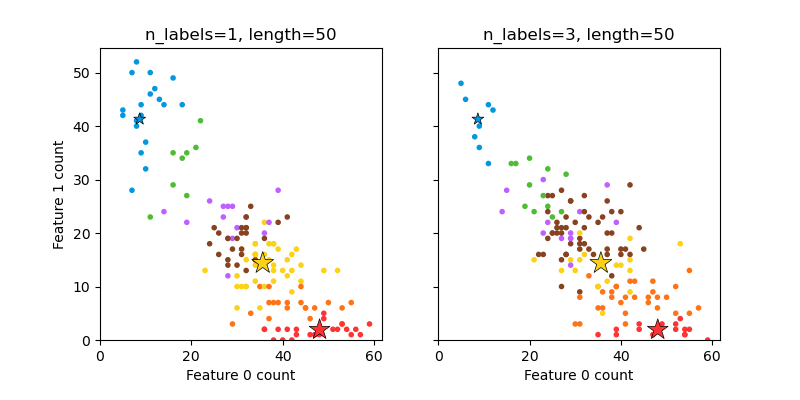# 7.3. Generated datasets¶

In addition, scikit-learn includes various random sample generators that can be used to build artificial datasets of controlled size and complexity.

## 7.3.1. Generators for classification and clustering¶

These generators produce a matrix of features and corresponding discrete targets.

### 7.3.1.1. Single label¶

Both make_blobs and make_classification create multiclass datasets by allocating each class one or more normally-distributed clusters of points. make_blobs provides greater control regarding the centers and standard deviations of each cluster, and is used to demonstrate clustering. make_classification specializes in introducing noise by way of: correlated, redundant and uninformative features; multiple Gaussian clusters per class; and linear transformations of the feature space.

make_gaussian_quantiles divides a single Gaussian cluster into near-equal-size classes separated by concentric hyperspheres. make_hastie_10_2 generates a similar binary, 10-dimensional problem.make_circles and make_moons generate 2d binary classification datasets that are challenging to certain algorithms (e.g. centroid-based clustering or linear classification), including optional Gaussian noise. They are useful for visualization. make_circles produces Gaussian data with a spherical decision boundary for binary classification, while make_moons produces two interleaving half circles.

### 7.3.1.2. Multilabel¶

make_multilabel_classification generates random samples with multiple labels, reflecting a bag of words drawn from a mixture of topics. The number of topics for each document is drawn from a Poisson distribution, and the topics themselves are drawn from a fixed random distribution. Similarly, the number of words is drawn from Poisson, with words drawn from a multinomial, where each topic defines a probability distribution over words. Simplifications with respect to true bag-of-words mixtures include:

• Per-topic word distributions are independently drawn, where in reality all would be affected by a sparse base distribution, and would be correlated.

• For a document generated from multiple topics, all topics are weighted equally in generating its bag of words.

• Documents without labels words at random, rather than from a base distribution.### 7.3.1.3. Biclustering¶

 make_biclusters(shape, n_clusters, *[, ...]) Generate a constant block diagonal structure array for biclustering. make_checkerboard(shape, n_clusters, *[, ...]) Generate an array with block checkerboard structure for biclustering.

## 7.3.2. Generators for regression¶

make_regression produces regression targets as an optionally-sparse random linear combination of random features, with noise. Its informative features may be uncorrelated, or low rank (few features account for most of the variance).

Other regression generators generate functions deterministically from randomized features. make_sparse_uncorrelated produces a target as a linear combination of four features with fixed coefficients. Others encode explicitly non-linear relations: make_friedman1 is related by polynomial and sine transforms; make_friedman2 includes feature multiplication and reciprocation; and make_friedman3 is similar with an arctan transformation on the target.

## 7.3.3. Generators for manifold learning¶

 make_s_curve([n_samples, noise, random_state]) Generate an S curve dataset. make_swiss_roll([n_samples, noise, ...]) Generate a swiss roll dataset.

## 7.3.4. Generators for decomposition¶

 make_low_rank_matrix([n_samples, ...]) Generate a mostly low rank matrix with bell-shaped singular values. make_sparse_coded_signal(n_samples, *, ...) Generate a signal as a sparse combination of dictionary elements. make_spd_matrix(n_dim, *[, random_state]) Generate a random symmetric, positive-definite matrix. make_sparse_spd_matrix([dim, alpha, ...]) Generate a sparse symmetric definite positive matrix.